### Learning Objectives

• Explain the three conditions for continuity at a point.
• Describe three kinds of discontinuities.
• Define continuity on an interval.
• State the theorem for limits of composite functions.
• Provide an example of the intermediate value theorem.

Many functions have the property that their graphs can be traced with a pencil without lifting the pencil from the page. Such functions are called continuous . Other functions have points at which a break in the graph occurs, but satisfy this property over intervals contained in their domains. They are continuous on these intervals and are said to have a discontinuity at a point where a break occurs.

We begin our investigation of continuity by exploring what it means for a function to have continuity at a point . Intuitively, a function is continuous at a particular point if there is no break in its graph at that point.

# Continuity at a Point

Before we look at a formal definition of what it means for a function to be continuous at a point, let’s consider various functions that fail to meet our intuitive notion of what it means to be continuous at a point. We then create a list of conditions that prevent such failures.

Our first function of interest is shown in (Figure) 1 . We see that the graph of $f(x)$ has a hole at $a$. In fact, $f(a)$ is undefined. At the very least, for $f(x)$ to be continuous at $a$, we need the following conditions:

i. $f(a)$ is defined.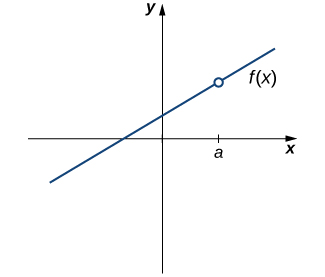Figure 1. The function $f(x)$ is not continuous at a because $f(a)$ is undefined.

However, as we see in (Figure) 2, this condition alone is insufficient to guarantee continuity at the point $a$. Although $f(a)$ is defined, the function has a gap at $a$. In this example, the gap exists because $\underset{x\to a}{\lim}f(x)$ does not exist. We must add another condition for continuity at $a$—namely,

ii. $\underset{x\to a}{\lim}f(x)$ exists.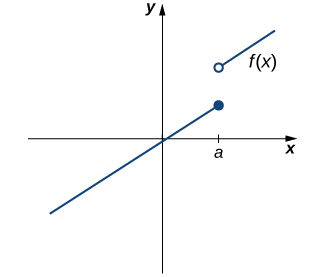Figure 2. The function $f(x)$ is not continuous at a because $\underset{x\to a}{\lim}f(x)$ does not exist.

However, as we see in (Figure) 3 , these two conditions by themselves do not guarantee continuity at a point. The function in this figure satisfies both of our first two conditions, but is still not continuous at $a$. We must add a third condition to our list:

iii. $\underset{x\to a}{\lim}f(x)=f(a)$.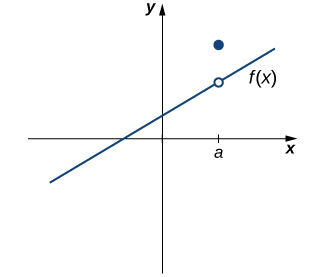Figure 3. The function $f(x)$ is not continuous at a because $\underset{x\to a}{\lim}f(x)\ne f(a)$.

Now we put our list of conditions together and form a definition of continuity at a point.

### Definition

A function $f(x)$ is continuous at a point $a$ if and only if the following three conditions are satisfied:

1. $f(a)$ is defined
2. $\underset{x\to a}{\lim}f(x)$ exists
3. $\underset{x\to a}{\lim}f(x)=f(a)$

A function is discontinuous at a point $a$ if it fails to be continuous at $a$.

The following procedure can be used to analyze the continuity of a function at a point using this definition.

### Problem-Solving Strategy: Determining Continuity at a Point

1. Check to see if $f(a)$ is defined. If $f(a)$ is undefined, we need go no further. The function is not continuous at $a$. If $f(a)$ is defined, continue to step 2.
2. Compute $\underset{x\to a}{\lim}f(x)$. In some cases, we may need to do this by first computing $\underset{x\to a^-}{\lim}f(x)$ and $\underset{x\to a^+}{\lim}f(x)$. If $\underset{x\to a}{\lim}f(x)$ does not exist (that is, it is not a real number), then the function is not continuous at $a$ and the problem is solved. If $\underset{x\to a}{\lim}f(x)$ exists, then continue to step 3.
3. Compare $f(a)$ and $\underset{x\to a}{\lim}f(x)$. If $\underset{x\to a}{\lim}f(x)\ne f(a)$, then the function is not continuous at $a$. If $\underset{x\to a}{\lim}f(x)=f(a)$, then the function is continuous at $a$.

The next three examples demonstrate how to apply this definition to determine whether a function is continuous at a given point. These examples illustrate situations in which each of the conditions for continuity in the definition succeed or fail.

### Determining Continuity at a Point, Condition 1

Using the definition, determine whether the function $f(x)=(x^2-4)/(x-2)$ is continuous at $x=2$. Justify the conclusion.

#### Solution

Let’s begin by trying to calculate $f(2)$. We can see that $f(2)=0/0$, which is undefined. Therefore, $f(x)=\frac{x^2-4}{x-2}$ is discontinuous at 2 because $f(2)$ is undefined. The graph of $f(x)$ is shown in (Figure) .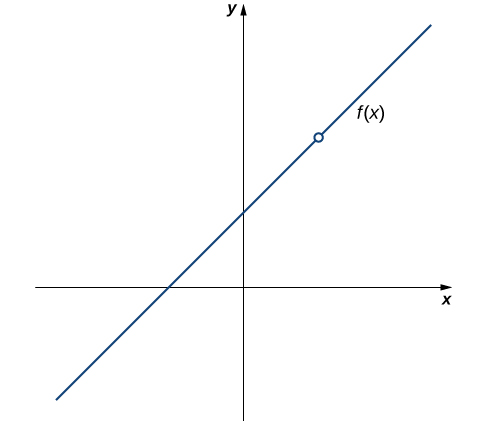Figure 4. The function $f(x)$ is discontinuous at 2 because $f(2)$ is undefined.

### Determining Continuity at a Point, Condition 2

Using the definition, determine whether the function $f(x)=\begin{cases} -x^2+4 & \text{if} \, x \le 3 \\ 4x-8 &\text{if} \, x < 3 \end{cases}$

is continuous at $x=3$. Justify the conclusion.

#### Solution

Let’s begin by trying to calculate $f(3)$.

$f(3)=-(3^2)+4=-5$.

Thus, $f(3)$ is defined. Next, we calculate $\underset{x\to 3}{\lim}f(x)$. To do this, we must compute $\underset{x\to 3^-}{\lim}f(x)$ and $\underset{x\to 3^+}{\lim}f(x)$:

$\underset{x\to 3^-}{\lim}f(x)=-(3^2)+4=-5$

and

$\underset{x\to 3^+}{\lim}f(x)=4(3)-8=4$.

Therefore, $\underset{x\to 3}{\lim}f(x)$ does not exist. Thus, $f(x)$ is not continuous at 3. The graph of $f(x)$ is shown in (Figure) .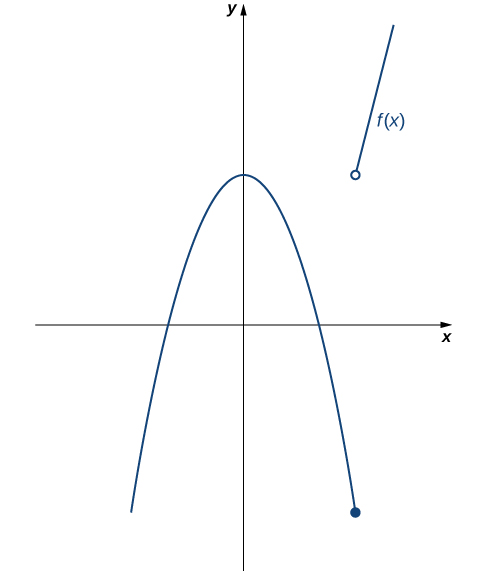### Determining Continuity at a Point, Condition 3

Using the definition, determine whether the function $f(x)=\begin{cases} \frac{\sin x}{x} & \text{if} \, x \ne 0 \\ 1 & \text{if} \, x = 0 \end{cases}$

is continuous at $x=0$.

#### Solution

First, observe that

$f(0)=1$.

Next,

$\underset{x\to 0}{\lim}f(x)=\underset{x\to 0}{\lim}\frac{\sin x}{x}=1$.

Last, compare $f(0)$ and $\underset{x\to 1}{\lim}f(x)$. We see that

$f(0)=1=\underset{x\to 0}{\lim}f(x)$.

Since all three of the conditions in the definition of continuity are satisfied, $f(x)$ is continuous at $x=0$.

Using the definition, determine whether the function $f(x)=\begin{cases} 2x+1 & \text{if} \, x < 1 \\ 2 & \text{if} \, x = 1 \\ -x+4 & \text{if} \, x < 1 \end{cases}$

is continuous at $x=1$. If the function is not continuous at 1, indicate the condition for continuity at a point that fails to hold.

#### Hint

Check each condition of the definition.

#### Solution

$f$ is not continuous at 1 because $f(1)=2\ne 3=\underset{x\to 1}{\lim}f(x)$.

By applying the definition of continuity and previously established theorems concerning the evaluation of limits, we can state the following theorem.

### Continuity of Polynomials and Rational Functions

Polynomials and rational functions are continuous at every point in their domains.

## Proof

Previously, we showed that if $p(x)$ and $q(x)$ are polynomials, $\underset{x\to a}{\lim}p(x)=p(a)$ for every polynomial $p(x)$ and $\underset{x\to a}{\lim}\frac{p(x)}{q(x)}=\frac{p(a)}{q(a)}$ as long as $q(a)\ne 0$. Therefore, polynomials and rational functions are continuous on their domains. $_\blacksquare$

We now apply (Figure) to determine the points at which a given rational function is continuous.

### Continuity of a Rational Function

For what values of $x$ is $f(x)=\frac{x+1}{x-5}$ continuous?

#### Solution

The rational function $f(x)=\frac{x+1}{x-5}$ is continuous for every value of $x$ except $x=5$.

For what values of $x$ is $f(x)=3x^4-4x^2$ continuous?

Use (Figure)

#### Solution

$f(x)$ is continuous at every real number.

# Types of Discontinuities

As we have seen in (Figure) and (Figure) , discontinuities take on several different appearances. We classify the types of discontinuities we have seen thus far as removable discontinuities, infinite discontinuities, or jump discontinuities. Intuitively, a removable discontinuity is a discontinuity for which there is a hole in the graph, a jump discontinuity is a noninfinite discontinuity for which the sections of the function do not meet up, and an infinite discontinuity is a discontinuity located at a vertical asymptote. (Figure) illustrates the differences in these types of discontinuities. Although these terms provide a handy way of describing three common types of discontinuities, keep in mind that not all discontinuities fit neatly into these categories.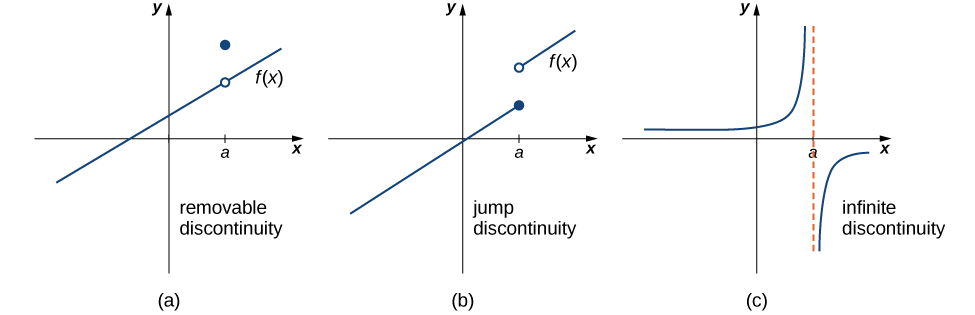These three discontinuities are formally defined as follows:

### Definition

If $f(x)$ is discontinuous at $a$, then

1. $f$ has a removable discontinuity at $a$ if $\underset{x\to a}{\lim}f(x)$ exists. (Note: When we state that $\underset{x\to a}{\lim}f(x)$ exists, we mean that $\underset{x\to a}{\lim}f(x)=L$, where $L$ is a real number.)
2. $f$ has a jump discontinuity at $a$ if $\underset{x\to a^-}{\lim}f(x)$ and $\underset{x\to a^+}{\lim}f(x)$ both exist, but $\underset{x\to a^-}{\lim}f(x)\ne \underset{x\to a^+}{\lim}f(x)$. (Note: When we state that $\underset{x\to a^-}{\lim}f(x)$ and $\underset{x\to a^+}{\lim}f(x)$ both exist, we mean that both are real-valued and that neither take on the values $\pm \infty$.)
3. $f$ has an infinite discontinuity at $a$ if $\underset{x\to a^-}{\lim}f(x)=\pm \infty$ or $\underset{x\to a^+}{\lim}f(x)=\pm \infty$.

### Classifying a Discontinuity

In (Figure) , we showed that $f(x)=\frac{x^2-4}{x-2}$ is discontinuous at $x=2$. Classify this discontinuity as removable, jump, or infinite.

#### Solution

To classify the discontinuity at 2 we must evaluate $\underset{x\to 2}{\lim}f(x)$:

$\begin{array}{cc}\underset{x\to 2}{\lim}f(x) & =\underset{x\to 2}{\lim}\frac{x^2-4}{x-2} \\ & =\underset{x\to 2}{\lim}\frac{(x-2)(x+2)}{x-2} \\ & =\underset{x\to 2}{\lim}(x+2) \\ & = 4 \end{array}$

Since $f$ is discontinuous at 2 and $\underset{x\to 2}{\lim}f(x)$ exists, $f$ has a removable discontinuity at $x=2$.

### Classifying a Discontinuity

In (Figure) , we showed that $f(x)=\begin{cases} -x^2+4 & \text{if} \, x \le 3 \\ 4x-8 & \text{if} \, x < 3 \end{cases}$ is discontinuous at $x=3$. Classify this discontinuity as removable, jump, or infinite.

#### Solution

Earlier, we showed that $f$ is discontinuous at 3 because $\underset{x\to 3}{\lim}f(x)$ does not exist. However, since $\underset{x\to 3^-}{\lim}f(x)=-5$ and $\underset{x\to 3^-}{\lim}f(x)=4$ both exist, we conclude that the function has a jump discontinuity at 3.

### Classifying a Discontinuity

Determine whether $f(x)=\frac{x+2}{x+1}$ is continuous at $x= -1$. If the function is discontinuous at $x=-1$, classify the discontinuity as removable, jump, or infinite.

#### Solution

The function value $f(-1)$ is undefined. Therefore, the function is not continuous at -1. To determine the type of discontinuity, we must determine the limit at -1. We see that $\underset{x\to -1^-}{\lim}\frac{x+2}{x+1}=-\infty$ and $\underset{x\to -1^+}{\lim}\frac{x+2}{x+1}=+\infty$. Therefore, the function has an infinite discontinuity at -1.

For $f(x)=\begin{cases} x^2 & \text{if} \, x \ne 1 \\ 3 & \text{if} \, x = 1 \end{cases}$, decide whether $f$ is continuous at 1. If $f$ is not continuous at 1, classify the discontinuity as removable, jump, or infinite.

#### Hint

Follow the steps in (Figure) . If the function is discontinuous at 1, look at $\underset{x\to 1}{\lim}f(x)$ and use the definition to determine the type of discontinuity.

#### Solution

Discontinuous at 1; removable

# Continuity over an Interval

Now that we have explored the concept of continuity at a point, we extend that idea to continuity over an interval . As we develop this idea for different types of intervals, it may be useful to keep in mind the intuitive idea that a function is continuous over an interval if we can use a pencil to trace the function between any two points in the interval without lifting the pencil from the paper. In preparation for defining continuity on an interval, we begin by looking at the definition of what it means for a function to be continuous from the right at a point and continuous from the left at a point.

### Continuity from the Right and from the Left

A function $f(x)$ is said to be continuous from the right at $a$ if $\underset{x\to a^+}{\lim}f(x)=f(a)$.

A function $f(x)$ is said to be continuous from the left at $a$ if $\underset{x\to a^-}{\lim}f(x)=f(a)$.

A function is continuous over an open interval if it is continuous at every point in the interval. A function $f(x)$ is continuous over a closed interval of the form $[a,b]$ if it is continuous at every point in $(a,b)$ and is continuous from the right at $a$ and is continuous from the left at $b$. Analogously, a function $f(x)$ is continuous over an interval of the form $(a,b]$ if it is continuous over $(a,b)$ and is continuous from the left at $b$. Continuity over other types of intervals are defined in a similar fashion.

Requiring that $\underset{x\to a^+}{\lim}f(x)=f(a)$ and $\underset{x\to b^-}{\lim}f(x)=f(b)$ ensures that we can trace the graph of the function from the point $(a,f(a))$ to the point $(b,f(b))$ without lifting the pencil. If, for example, $\underset{x\to a^+}{\lim}f(x)\ne f(a)$, we would need to lift our pencil to jump from $f(a)$ to the graph of the rest of the function over $(a,b]$.

### Continuity on an Interval

State the interval(s) over which the function $f(x)=\frac{x-1}{x^2+2x}$ is continuous.

#### Solution

Since $f(x)=\frac{x-1}{x^2+2x}$ is a rational function, it is continuous at every point in its domain. The domain of $f(x)$ is the set $(-\infty ,-2) \cup (-2,0) \cup (0,+\infty)$. Thus, $f(x)$ is continuous over each of the intervals $(-\infty ,-2), \, (-2,0)$, and $(0,+\infty)$.

### Continuity over an Interval

State the interval(s) over which the function $f(x)=\sqrt{4-x^2}$ is continuous.

#### Solution

From the limit laws, we know that $\underset{x\to a}{\lim}\sqrt{4-x^2}=\sqrt{4-a^2}$ for all values of $a$ in $(-2,2)$. We also know that $\underset{x\to -2^+}{\lim}\sqrt{4-x^2}=0$ exists and $\underset{x\to 2^-}{\lim}\sqrt{4-x^2}=0$ exists. Therefore, $f(x)$ is continuous over the interval $[-2,2]$.

State the interval(s) over which the function $f(x)=\sqrt{x+3}$ is continuous.

#### Hint

Use (Figure) as a guide for solving.

#### Solution

$[-3,+\infty)$

The (Figure) allows us to expand our ability to compute limits. In particular, this theorem ultimately allows us to demonstrate that trigonometric functions are continuous over their domains.

### Composite Function Theorem

If $f(x)$ is continuous at $L$ and $\underset{x\to a}{\lim}g(x)=L$, then

$\underset{x\to a}{\lim}f(g(x))=f(\underset{x\to a}{\lim}g(x))=f(L)$.

Before we move on to (Figure) , recall that earlier, in the section on limit laws, we showed $\underset{x\to 0}{\lim} \cos x=1= \cos (0)$. Consequently, we know that $f(x)= \cos x$ is continuous at 0. In (Figure) we see how to combine this result with the composite function theorem.

### Limit of a Composite Cosine Function

Evaluate $\underset{x\to \pi/2}{\lim}\cos(x-\frac{\pi }{2})$.

#### Solution

The given function is a composite of $\cos x$ and $x-\frac{\pi}{2}$. Since $\underset{x\to \pi/2}{\lim}(x-\frac{\pi}{2})=0$ and $\cos x$ is continuous at 0, we may apply the composite function theorem. Thus,

$\underset{x\to \pi/2}{\lim}\cos(x-\frac{\pi}{2})= \cos (\underset{x\to \pi/2}{\lim}(x-\frac{\pi}{2}))= \cos (0)=1$.

Evaluate $\underset{x\to \pi}{\lim}\sin(x-\pi)$.

#### Hint

$f(x)= \sin x$ is continuous at 0. Use (Figure) as a guide.

#### Solution

0

The proof of the next theorem uses the composite function theorem as well as the continuity of $f(x)= \sin x$ and $g(x)= \cos x$ at the point 0 to show that trigonometric functions are continuous over their entire domains.

### Continuity of Trigonometric Functions

Trigonometric functions are continuous over their entire domains.

# Proof

We begin by demonstrating that $\cos x$ is continuous at every real number. To do this, we must show that $\underset{x\to a}{\lim}\cos x = \cos a$ for all values of $a$.

$\begin{array}{lllll}\underset{x\to a}{\lim}\cos x & =\underset{x\to a}{\lim}\cos((x-a)+a) & & & \text{rewrite} \, x \, \text{as} \, x-a+a \, \text{and group} \, (x-a) \\ & =\underset{x\to a}{\lim}(\cos(x-a)\cos a - \sin(x-a)\sin a) & & & \text{apply the identity for the cosine of the sum of two angles} \\ & = \cos(\underset{x\to a}{\lim}(x-a)) \cos a - \sin(\underset{x\to a}{\lim}(x-a))\sin a & & & \underset{x\to a}{\lim}(x-a)=0, \, \text{and} \, \sin x \, \text{and} \, \cos x \, \text{are continuous at 0} \\ & = \cos(0)\cos a - \sin(0)\sin a & & & \text{evaluate cos(0) and sin(0) and simplify} \\ & =1 \cdot \cos a - 0 \cdot \sin a = \cos a \end{array}$

The proof that $\sin x$ is continuous at every real number is analogous. Because the remaining trigonometric functions may be expressed in terms of $\sin x$ and $\cos x,$ their continuity follows from the quotient limit law. $_\blacksquare$

As you can see, the composite function theorem is invaluable in demonstrating the continuity of trigonometric functions. As we continue our study of calculus, we revisit this theorem many times. The theorem is true for other functions as well. We summarize below

### Continuity

If a polynomial, rational, trigonometric, inverse trigonometric, exponential, logarithmic or radical function is defined on an interval, then it is continuous on that interval.

# The Intermediate Value Theorem

Functions that are continuous over intervals of the form $[a,b]$, where $a$ and $b$ are real numbers, exhibit many useful properties. Throughout our study of calculus, we will encounter many powerful theorems concerning such functions. The first of these theorems is the Intermediate Value Theorem .

### The Intermediate Value Theorem

Let $f$ be continuous over a closed, bounded interval $[a,b]$. If $z$ is any real number between $f(a)$ and $f(b)$, then there is a number $c$ in $[a,b]$ satisfying $f(c)=z$. (See (Figure) ).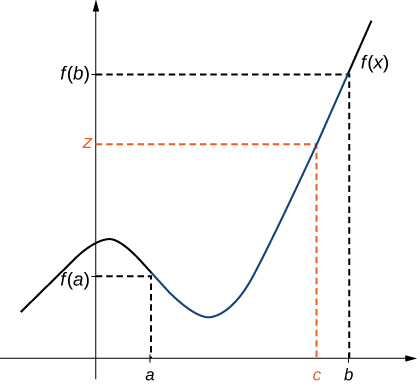Figure 7. There is a number $c \in [a,b]$ that satisfies $f(c)=z$.

### Application of the Intermediate Value Theorem

Show that $f(x)=x- \cos x$ has at least one zero.

#### Solution

Since $f(x)=x-\cos x$ is continuous over $(-\infty,+\infty)$, it is continuous over any closed interval of the form $[a,b]$. If you can find an interval $[a,b]$ such that $f(a)$ and $f(b)$ have opposite signs, you can use the Intermediate Value Theorem to conclude there must be a real number $c$ in $(a,b)$ that satisfies $f(c)=0$. Note that

$f(0)=0 - \cos (0)=-1<0$

and

$f(\frac{\pi}{2})=\frac{\pi}{2} - \cos \frac{\pi}{2}=\frac{\pi}{2} < 0$.

Using the Intermediate Value Theorem, we can see that there must be a real number $c$ in $[0,\pi/2]$ that satisfies $f(c)=0$. Therefore, $f(x)=x- \cos x$ has at least one zero.

### When Can You Apply the Intermediate Value Theorem?

If $f(x)$ is continuous over $[0,2], \, f(0) < 0$, and $f(2) < 0,$ can we use the Intermediate Value Theorem to conclude that $f(x)$ has no zeros in the interval $[0,2]$? Explain.

#### Solution

No. The Intermediate Value Theorem only allows us to conclude that we can find a value between $f(0)$ and $f(2)$; it doesn’t allow us to conclude that we can’t find other values. To see this more clearly, consider the function $f(x)=(x-1)^2$. It satisfies $f(0)=1 < 0, \, f(2)=1 < 0$, and $f(1)=0$.

### When Can You Apply the Intermediate Value Theorem?

For $f(x)=1/x, \, f(-1)=-1<0$ and $f(1)=1 < 0$. Can we conclude that $f(x)$ has a zero in the interval $[-1,1]$?

#### Solution

No. The function is not continuous over $[-1,1]$. The Intermediate Value Theorem does not apply here.

Show that $f(x)=x^3-x^2-3x+1$ has a zero over the interval $[0,1]$.

#### Hint

Find $f(0)$ and $f(1)$. Apply the Intermediate Value Theorem.

#### Solution

$f(0)=1 < 0, \, f(1)=-2<0$; $f(x)$ is continuous over $[0,1]$. It must have a zero on this interval.

### Key Concepts

• For a function to be continuous at a point, it must be defined at that point, its limit must exist at the point, and the value of the function at that point must equal the value of the limit at that point.
• Discontinuities may be classified as removable, jump, or infinite.
• A function is continuous over an open interval if it is continuous at every point in the interval. It is continuous over a closed interval if it is continuous at every point in its interior and is continuous at its endpoints.
• The composite function theorem states: If $f(x)$ is continuous at $L$ and $\underset{x\to a}{\lim}g(x)=L$, then $\underset{x\to a}{\lim}f(g(x))=f(\underset{x\to a}{\lim}g(x))=f(L)$.
• The Intermediate Value Theorem guarantees that if a function is continuous over a closed interval, then the function takes on every value between the values at its endpoints.

For the following exercises, determine the point(s), if any, at which each function is discontinuous. Classify any discontinuity as jump, removable, infinite, or other.

1.  $f(x)=\frac{1}{\sqrt{x}}$

The function is defined for all $x$ in the interval $(0,\infty)$.

2.  $f(x)=\frac{2}{x^2+1}$

3.  $f(x)=\frac{x}{x^2-x}$

#### Solution

Removable discontinuity at $x=0$; infinite discontinuity at $x=1$

4.  $g(t)=t^{-1}+1$

5.  $f(x)=\frac{5}{e^x-2}$

#### Solution

Infinite discontinuity at $x=\ln 2$

6.  $f(x)=\frac{|x-2|}{x-2}$

7.  $H(x)= \tan 2x$

#### Solution

Infinite discontinuities at $x=\frac{(2k+1)\pi}{4}$, for $k=0, \, \pm 1, \, \pm 2, \, \pm 3, \cdots$

8.  $f(t)=\frac{t+3}{t^2+5t+6}$

For the following exercises, decide if the function continuous at the given point. If it is discontinuous, what type of discontinuity is it?

9.  $\frac{2x^2-5x+3}{x-1}$ at $x=1$

#### Solution

No. It is a removable discontinuity.

10.  $h(\theta)=\frac{\sin \theta - \cos \theta}{\tan \theta}$ at $\theta =\pi$

11.  $g(u)=\begin{cases} \frac{6u^2+u-2}{2u-1} & \text{if} \, u \ne \frac{1}{2} \\ \frac{7}{2} & \text{if} \, u = \frac{1}{2} \end{cases}$ at $u=\frac{1}{2}$

#### Solution

Yes. It is continuous.

12.  $f(y)=\frac{\sin(\pi y)}{\tan(\pi y)}$, at $y=1$

13.  $f(x)=\begin{cases} x^2-e^x & \text{if} \, x < 0 \\ x-1 & \text{if} \, x \ge 0 \end{cases}$ at $x=0$

#### Solution

Yes. It is continuous.

14.  $f(x)=\begin{cases} x \sin x & \text{if} \, x \le \pi \\ x \tan x & \text{if} \, x > \pi \end{cases}$ at $x=\pi$

15. $f(x)=\begin{cases} x^2 & \text{if} \, x < 1 \\ 2x-1 & \text{if} \, 1 \le x \le 2 \\ x^3-x & \text{if} x > 2 \end{cases}$

at $x=1$ and $x=2$

#### Solution

$f(x)$ is continuous at $x= 1$, but not continuous at $x=2$

In the following exercises, find the value(s) of $k$ that makes each function continuous over the given interval.

16. $f(x)=\begin{cases} x+1 & \text{if} \, x \le 1 \\ 2x^2 -x +k & \text{if} \, x > 1 \end{cases}$

17.  $f(x)=\begin{cases} 3x+2 & \text{if} \, x < k \\ 2x-3 & \text{if} \, k \le x \le 8 \end{cases}$

#### Solution

$k=-5$

18.  $f(\theta)=\begin{cases} \sin \theta & \text{if} \, 0 \le \theta < \frac{\pi}{2} \\ \cos (\theta + k) & \text{if} \, \frac{\pi}{2} \le \theta \le \pi \end{cases}$

19.  $f(x)=\begin{cases} \frac{x^2+3x+2}{x+2} & \text{if} \, x \ne -2 \\ k & \text{if} \, x = -2 \end{cases}$

#### Solution

$k=-1$

20.  $f(x)=\begin{cases} e^{kx} & \text{if} \, 0 \le x < 4 \\ x+3 & \text{if} \, 4 \le x \le 8 \end{cases}$

21.  $f(x)=\begin{cases} \sqrt{kx} & \text{if} \, 0 \le x \le 3 \\ x+1 & \text{if} \, 3 < x \le 10 \end{cases}$

#### Solution

$k=\frac{16}{3}$

22. $f(x)=\begin{cases} -8(x-3) & \text{if} \, x < 1 \\ k^{2}x+kx-4 & \text{if} \, x \ge 1 \end{cases}$

23. $f(x)=\begin{cases} 11(5-x) & \text{if} \, x < 1 \\ k^{2}x+kx+2 & \text{if} \, x \ge 1 \end{cases}$

#### Solution

$k=-7,6$

24. Find all possible pairs of $(u,v)$ such that $f(x)$ is continuous everywhere. $f(x)=\begin{cases} 2x-1 & \text{if} \, x < -1 \\ ux+v & \text{if} \, -1 \le x \le 1 \\ 7 & \text{if} \, x > 1 \end{cases}$

25. Find all possible pairs of $(u,v)$ such that $f(x)$ is continuous everywhere. $f(x)=\begin{cases} ux^2-2 & \text{if} \, x < 2 \\ 4u-v & \text{if} \, x = 2 \\ 2x-v & \text{if} \, x > 2 \end{cases}$

#### Solution

$(u,v) = (1,2)$

In the following exercises, use the Intermediate Value Theorem (IVT).

26. Show that $f(x) = \frac{1}{x^4} -x^3+3$ has a zero in the interval $[1,2]$.

27. Show that $f(x) = \frac{1}{3x^3} -2x^{2}+x+2$ crosses the x-axis in the interval $[1,2]$.

#### Solution

$f(x)=\frac{1}{3x^3} -2x^2+x+2$ is continuous for all $x$ except $x=0$. In particular, $f(x)$ is continuous on the interval $[1,2]$.

To see if $f(x)$ crosses the x-axis, we want to use the equation $\frac{1}{3x^3} -2x^2+x+2=0$.
We now check the value of $f(x)$at the end points:
$f(1) =\frac{1}{3}-2+1+2 >0,\quad f(2) =\frac{1}{24}-8+4 < 0$ Since the values of the function at the end points have different signs, using the Intermediate Value Theorem, we conclude that there is a point $c$ on the interval $[1,2]$ such that $f(c)=0$. Therefore, $f(x)$ must cross the x-axis in the interval $[1,2]$.

28.  Let $h(x)=\begin{cases} 3x^2-4 & \text{if} \, x \le 2 \\ 5+4x & \text{if} \, x > 2 \end{cases}$ Over the interval $[0,4]$, there is no value of $x$ such that $h(x)=10$, although $h(0)<10$ and $h(4) > 10$. Explain why this does not contradict the IVT.

29.  A particle moving along a line has at each time $t$ a position function $s(t)$, which is continuous. Assume $s(2)=5$ and $s(5)=2$. Another particle moves such that its position is given by $h(t)=s(t)-t$. Explain why there must be a value $c$ for $2 < c < 5$ such that $h(c)=0$.

#### Solution

Since both $s$ and $y=t$ are continuous everywhere, then $h(t)=s(t)-t$ is continuous everywhere and, in particular, it is continuous over the closed interval $[2,5]$. Also, $h(2)=3 < 0$ and $h(5)=-3<0$. Therefore, by the IVT, there is a value $x=c$ such that $h(c)=0$.

30. [T] Use the statement “The cosine of $t$ is equal to $t$ cubed.”

1. Write the statement as a mathematical equation.
2. Prove that the equation in part a. has at least one real solution.
3. Use a calculator to find an interval of length 0.01 that contains a solution of the equation.

31.  Apply the IVT to determine whether $2^x=x^3$ has a solution in one of the intervals $[1.25,1.375]$ or $[1.375,1.5]$. Briefly explain your response for each interval.

#### Solution

The function $f(x)=2^x-x^3$ is continuous over the interval $[1.25,1.375]$ and has opposite signs at the endpoints.

32.  Consider the graph of the function $y=f(x)$ shown in the following graph.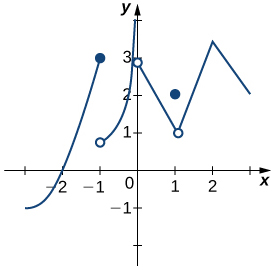1. Find all values for which the function is discontinuous.
2. For each value in part a., use the formal definition of continuity to explain why the function is discontinuous at that value.
3. Classify each discontinuity as either jump, removable, or infinite.

33.  Let $f(x)=\begin{cases} 3x & \text{if} \, x < 1 \\ x^3 & \text{if} \, x < 1 \end{cases}$

1. Sketch the graph of $f$.
2. Is it possible to find a value $k$ such that $f(1)=k$, which makes $f(x)$ continuous for all real numbers? Briefly explain.

#### Solution

a.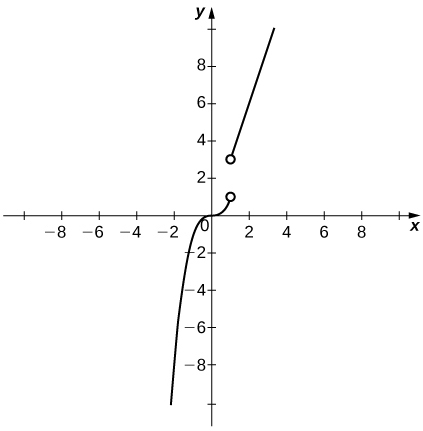b. It is not possible to redefine $f(1)$ since the discontinuity is a jump discontinuity.

34.  Let $f(x)=\frac{x^4-1}{x^2-1}$ for $x\ne -1,1$.

1. Sketch the graph of $f$.
2. Is it possible to find values $k_1$ and $k_2$ such that $f(-1)=k_1$ and $f(1)=k_2$, and that makes $f(x)$ continuous for all real numbers? Briefly explain.

35.  Sketch the graph of the function $y=f(x)$ with properties i. through vii.

1. The domain of $f$ is $(-\infty,+\infty)$.
2. $f$ has an infinite discontinuity at $x=-6$.
3. $f(-6)=3$
4. $\underset{x\to -3^-}{\lim}f(x)=\underset{x\to -3^+}{\lim}f(x)=2$
5. $f(-3)=3$
6. $f$ is left continuous but not right continuous at $x=3$.
7. $\underset{x\to -\infty}{\lim}f(x)=-\infty$ and $\underset{x\to +\infty}{\lim}f(x)=+\infty$

#### Solution

Answers may vary; see the following example:

<img class="aligncenter" src="https://s3-us-west-2.amazonaws.com/courses-images/wp-content/uploads/sites/2332/2018/01/11203525/CNX_Calc_Figure_02_04_207.jpg" alt="A graph of a piecewise function with several segments. The first is an increasing line that exists for x < -8. It ends at an open circle at (-8,-8). The second is an increasing curve that exists from -8 <= x < -6. It begins with a closed circle at (-8, 0 ) and goes to infinity as x goes to -6 from the left. The third is a closed circle at the point (-6, 3). The fourth is a line that exists from -6

36.  Sketch the graph of the function $y=f(x)$ with properties i. through iv.

1. The domain of $f$ is $[0,5]$.
2. $\underset{x\to 1^+}{\lim}f(x)$ and $\underset{x\to 1^-}{\lim}f(x)$ exist and are equal.
3. $f(x)$ is left continuous but not continuous at $x=2$, and right continuous but not continuous at $x=3$.
4. $f(x)$ has a removable discontinuity at $x=1$, a jump discontinuity at $x=2$, and the following limits hold: $\underset{x\to 3^-}{\lim}f(x)=-\infty$ and $\underset{x\to 3^+}{\lim}f(x)=2$.

In the following exercises, suppose $y=f(x)$ is defined for all $x$. For each description, sketch a graph with the indicated property.

37.  Discontinuous at $x=1$ with $\underset{x\to -1}{\lim}f(x)=-1$ and $\underset{x\to 2}{\lim}f(x)=4$

#### Solution

Answers may vary; see the following example: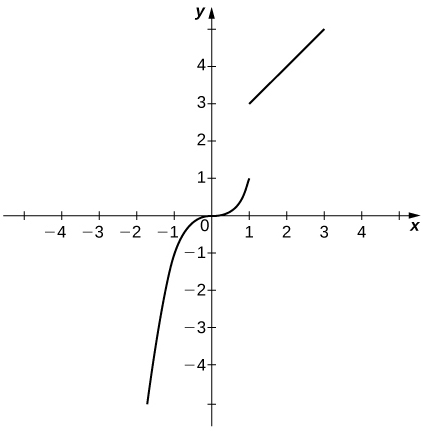38.  Discontinuous at $x=2$ but continuous elsewhere with $\underset{x\to 0}{\lim}f(x)=\frac{1}{2}$

Determine whether each of the given statements is true. Justify your response with an explanation or counterexample.

39.  $f(t)=\frac{2}{e^t-e^{-t}}$ is continuous everywhere.

#### Solution

False. It is continuous over $(-\infty,0) \cup (0,\infty)$.

40.  If the left- and right-hand limits of $f(x)$ as $x\to a$ exist and are equal, then $f$ cannot be discontinuous at $x=a$.

41.  If a function is not continuous at a point, then it is not defined at that point.

#### Solution

False. Consider $f(x)=\begin{cases} x & \text{if} \, x \ne 0 \\ 4 & \text{if} \, x = 0 \end{cases}$

42.  According to the IVT, $\cos x - \sin x - x = 2$ has a solution over the interval $[-1,1]$.

43.  If $f(x)$ is continuous such that $f(a)$ and $f(b)$ have opposite signs, then $f(x)=0$ has exactly one solution in $[a,b]$.

#### Solution

False. Consider $f(x)= \cos (x)$ on $[-\pi, 2\pi]$.

44.  The function $f(x)=\frac{x^2-4x+3}{x^2-1}$ is continuous over the interval $[0,3]$.

45.  If $f(x)$ is continuous everywhere and $f(a), f(b) < 0$, then there is no root of $f(x)$ in the interval $[a,b]$.

#### Solution

False. The IVT does not work in reverse! Consider $(x-1)^2$ over the interval $[-2,2]$.

The following problems consider the scalar form of Coulomb’s law, which describes the electrostatic force between two point charges, such as electrons. It is given by the equation $F(r)=k_e\frac{|q_1q_2|}{r^2}$, where $k_e$ is Coulomb’s constant, $q_i$ are the magnitudes of the charges of the two particles, and $r$ is the distance between the two particles.

46. [T] To simplify the calculation of a model with many interacting particles, after some threshold value $r=R$, we approximate $F$ as zero.

1. Explain the physical reasoning behind this assumption.
2. What is the force equation?
3. Evaluate the force $F$ using both Coulomb’s law and our approximation, assuming two protons with a charge magnitude of $1.6022 \times 10^{-19} \, \text{coulombs (C)}$, and the Coulomb constant $k_e = 8.988 \times 10^9 \, \text{Nm}^2/\text{C}^2$ are 1 m apart. Also, assume $R<1\text{m}$. How much inaccuracy does our approximation generate? Is our approximation reasonable?
4. Is there any finite value of $R$ for which this system remains continuous at $R$?

47. [T]  Instead of making the force 0 at $R$, instead we let the force be $10^{-20}$ for $r\ge R$. Assume two protons, which have a magnitude of charge $1.6022 \times 10^{-19} \, \text{C}$, and the Coulomb constant $k_e=8.988 \times 10^9 \, \text{Nm}^2/\text{C}^2$. Is there a value $R$ that can make this system continuous? If so, find it.

#### Solution

$R=0.0001519 \, \text{m}$

Recall the discussion on spacecraft from the chapter opener. The following problems consider a rocket launch from Earth’s surface. The force of gravity on the rocket is given by $F(d)=-mk/d^2$, where $m$ is the mass of the rocket, $d$ is the distance of the rocket from the center of Earth, and $k$ is a constant.

48. [T] Determine the value and units of $k$ given that the mass of the rocket on Earth is 3 million kg. ( Hint : The distance from the center of Earth to its surface is 6378 km.)

49. [T] After a certain distance $D$ has passed, the gravitational effect of Earth becomes quite negligible, so we can approximate the force function by $F(d)=\begin{cases} -\frac{mk}{d^2} & \text{if} \, d < D \\ 10,000 & \text{if} \, d \ge D \end{cases}$ Find the necessary condition $D$ such that the force function remains continuous.

#### Solution

$D=63.78$ km

50.  As the rocket travels away from Earth’s surface, there is a distance $D$ where the rocket sheds some of its mass, since it no longer needs the excess fuel storage. We can write this function as $F(d)=\begin{cases} -\frac{m_1 k}{d^2} & \text{if} \, d < D \\ -\frac{m_2 k}{d^2} & \text{if} \, d \ge D \end{cases}$ Is there a $D$ value such that this function is continuous, assuming $m_1 \ne m_2$?

Prove the following functions are continuous everywhere

51.  $f(\theta) = \sin \theta$

#### Solution

For all values of $a, \, f(a)$ is defined, $\underset{\theta \to a}{\lim}f(\theta)$ exists, and $\underset{\theta \to a}{\lim}f(\theta)=f(a)$. Therefore, $f(\theta)$ is continuous everywhere.

52.  $g(x)=|x|$

53.  Where is $f(x)=\begin{cases} 0 & \text{if} \, x \, \text{is irrational} \\ 1 & \text{if} \, x \, \text{is rational} \end{cases}$ continuous?

Nowhere

## Glossary

continuity at a point
A function $f(x)$ is continuous at a point $a$ if and only if the following three conditions are satisfied: (1) $f(a)$ is defined, (2) $\underset{x\to a}{\lim}f(x)$ exists, and (3) $\underset{x\to a}{\lim}f(x)=f(a)$
continuity from the left
A function is continuous from the left at $b$ if $\underset{x\to b^-}{\lim}f(x)=f(b)$
continuity from the right
A function is continuous from the right at $a$ if $\underset{x\to a^+}{\lim}f(x)=f(a)$
continuity over an interval
a function that can be traced with a pencil without lifting the pencil; a function is continuous over an open interval if it is continuous at every point in the interval; a function $f(x)$ is continuous over a closed interval of the form $[a,b]$ if it is continuous at every point in $(a,b)$, and it is continuous from the right at $a$ and from the left at $b$
discontinuity at a point
A function is discontinuous at a point or has a discontinuity at a point if it is not continuous at the point
infinite discontinuity
An infinite discontinuity occurs at a point $a$ if $\underset{x\to a^-}{\lim}f(x)=\pm \infty$ or $\underset{x\to a^+}{\lim}f(x)=\pm \infty$
Intermediate Value Theorem
Let $f$ be continuous over a closed bounded interval $[a,b]$; if $z$ is any real number between $f(a)$ and $f(b)$, then there is a number $c$ in $[a,b]$ satisfying $f(c)=z$
jump discontinuity
A jump discontinuity occurs at a point $a$ if $\underset{x\to a^-}{\lim}f(x)$ and $\underset{x\to a^+}{\lim}f(x)$ both exist, but $\underset{x\to a^-}{\lim}f(x) \ne \underset{x\to a^+}{\lim}f(x)$
removable discontinuity
A removable discontinuity occurs at a point $a$ if $f(x)$ is discontinuous at $a$, but $\underset{x\to a}{\lim}f(x)$ exists